• 报错：scanf_s函数报错:没有为格式字符串传递足够的参数 这个问题很变态 解决办法：在使用%c和%s读入字符或字符串时，应在地址参数后附加一个缓冲区边界值。 int main() { char s1 = "i m name"; char *...
报错：scanf_s函数报错:没有为格式字符串传递足够的参数

int main() {
char s1 = "i m name";

char *p1, *p2;
char s3 = {0};

p1 = s1;
p2 = s3;
printf("请输入你的名字: \n");
scanf_s("%s",&s3);

printf("%s\n", s3);

for (; *p1 != '\0'; p1++) {
if (*p1 == *p2) {
p2 = s3;
}
printf("I am %s\n", s3);
}

while (true){}
return 0;
}


这个问题很变态

解决办法：在使用%c和%s读入字符或字符串时，应在地址参数后附加一个缓冲区边界值。

int main() {
char s1 = "i m name";

char *p1, *p2;
char s3 = {0};

p1 = s1;
p2 = s3;
printf("请输入你的名字: \n");
//scanf_s("%s",&s3);
scanf_s("%s",&s3,sizeof(s3));   //使用sizeof

printf("%s\n", s3);

for (; *p1 != '\0'; p1++) {
if (*p1 == *p2) {
p2 = s3;
}
printf("I am %s\n", s3);
}

while (true){}
return 0;
}


总结;就会提示你没有为格式字符串传递足够的参数。
首先我们来看一下scanf和scanf_s的区别
scanf()不会检查输入边界，可能造成数据溢出。
scanf_s()会进行边界检查。
scanf()函数是标准C中提供的标准输入函数，用以用户输入数据
scanf_s()函数是Microsoft公司VS开发工具提供的一个功能相同的安全标准输入函数，从vc++2005开始，VS系统提供了scanf_s()。在调用该函数时，必须提供一个数字以表明最多读取多少位字符。

上面的程序有点复杂;下面来一个简单点的例子：

int main(void) {
char str1 = { 0 };
char str2 = { 0 };
char str3 = { 0 };
//gets() 用法
printf("Input a string: ");
gets_s(str1);
//scanf() 用法
printf("Input a string: ");
scanf_s("%s", str2);
scanf_s("%s", str3);

printf("\nstr1: %s\n", str1);
printf("str2: %s\n", str2);
printf("str3: %s\n", str3);
while (true){}
return 0;
}


上面的代码当你输入字符串的时候会报错：没有为格式字符串传递足够的参数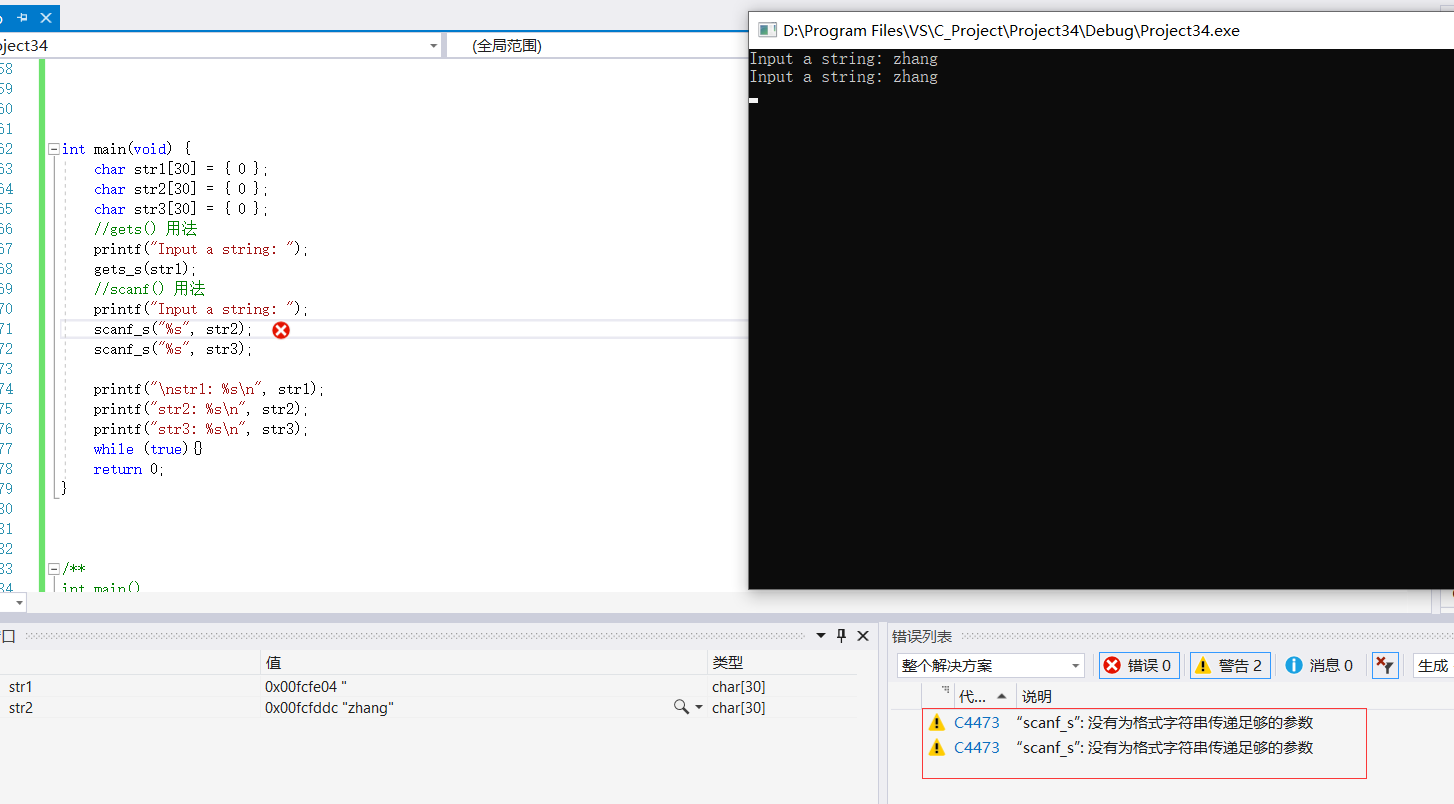解决办法：在使用%c和%s读入字符或字符串时，应在地址参数后附加一个缓冲区边界值。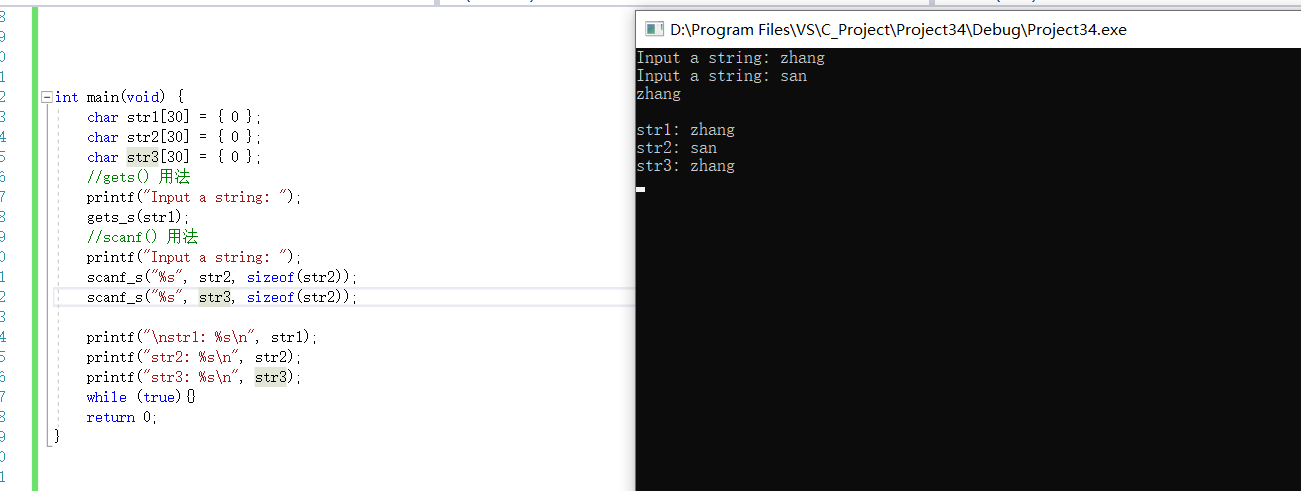展开全文• ## s型函数

千次阅读 2019-10-01 11:42:40
S函数 性质1：导数最大值的求解 f(x)=A1+ea−bxf(x)=\frac{A}{1+e^{a-bx}}f(x)=1+ea−bxA​ 性质2：中心对称的证明 对f(x)f(x)f(x)求导的dfdx=Abea−bx(ea−bx+1)2=Ab[(ea−bx)12+(ea−bx)−12]2\frac{df}{dx}=\...
性质1：导数最大值的求解
$f(x)=\frac{A}{1+e^{a-bx}}$
对$f(x)$求导的$\frac{df}{dx}=\frac{Abe^{a-bx}}{(e^{a-bx}+1)^2}=\frac{Ab}{[(e^{a-bx})^\frac{1}{2}+(e^{a-bx})^{-\frac{1}{2}}]^2}$
由$(e^{a-bx})^{\frac{1}{2}}\ge0$和$(e^{a-bx})^{-\frac{1}{2}}\ge0$可以满足以下条件:
$(e^{a-bx})^{1/2}+（e^{a-bx})^{-1/2}\ge \sqrt{(e^{a-bx})^{1/2}\times(e^{a-bx})^{-1/2}}=2$当$(e^{a-bx})^{1/2}=(e^{a-bx})^{-1/2}$时取得$"="$
所以$(e^{a-bx})^{1/2}+(e^{a-bx})^{-1/2}$最小值为$2$
$f'(x)=\frac{Ab}{[(e^{a-bx})^{1/2}+(e^{a-bx})^{-1/2}]}\leq{ \frac{Ab}{4}}$
性质2：中心对称的证明
关于点$(x_0,y_0)$中心对称函数满足性质$f(x_0+x)+f(x_0-x)=2y_0$
假设$s$型函数的中心对称，且关于点$(\frac{a}{b},\frac{A}{2})$中心对称则满足
$f(\frac{a}{b}+x)+f(\frac{a}{b}-x)=\frac{A}{1+e^{-bx}}=A……（1）$
易得
$f(\frac{a}{b}+x)+\frac{A}{1+e^{a-b(\frac{a}{b}+x)}}=\frac{A}{1+e^{-bx}}=\frac{Ae^{bx}}{1+e^{bx}}……(2)$
$f(\frac{a}{b}-x)+\frac{A}{1+e^{a-b(\frac{a}{b}-x)}}=\frac{A}{1+e^{bx}}……（3）$
由（2）和（3）式子的（1）式成立
既：s型函数关于点$(\frac{a}{b},\frac{A}{2})$对称
性质3：经过定点 $(0,\frac{A}{1+e^a})$
当多组$f(x)=\frac{A}{1+e^{a-bx}}$函数中参数A,a相同参数b不同则函数同过点$(0,\frac{A}{1+e^a})$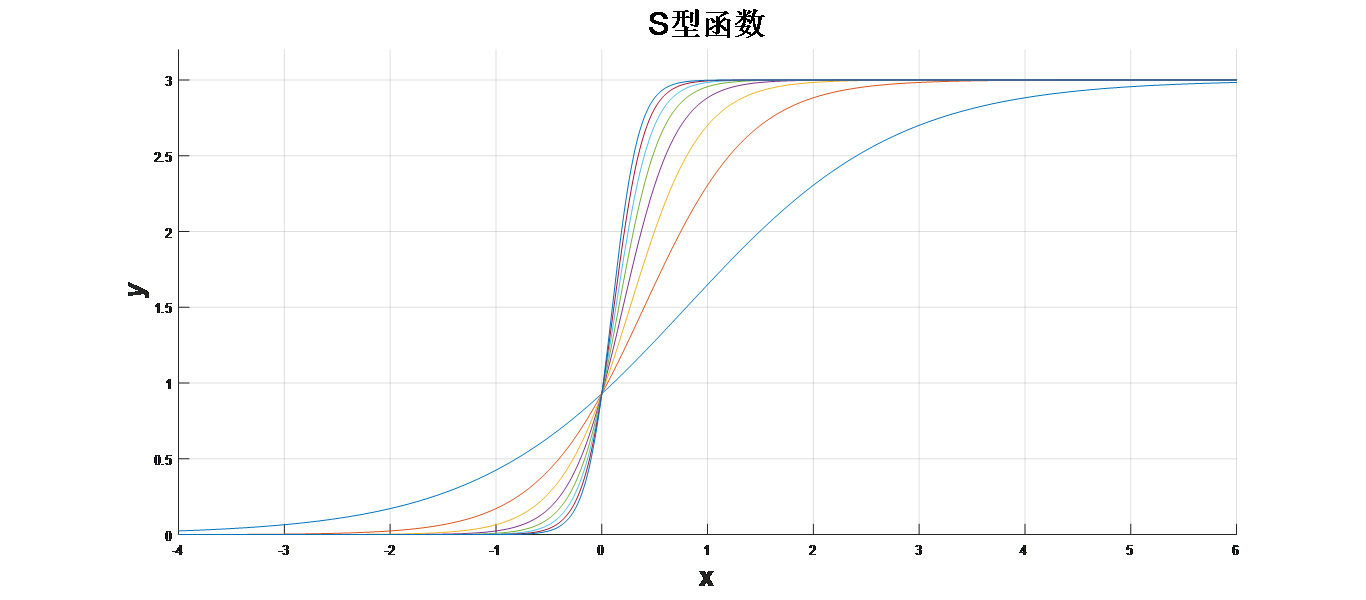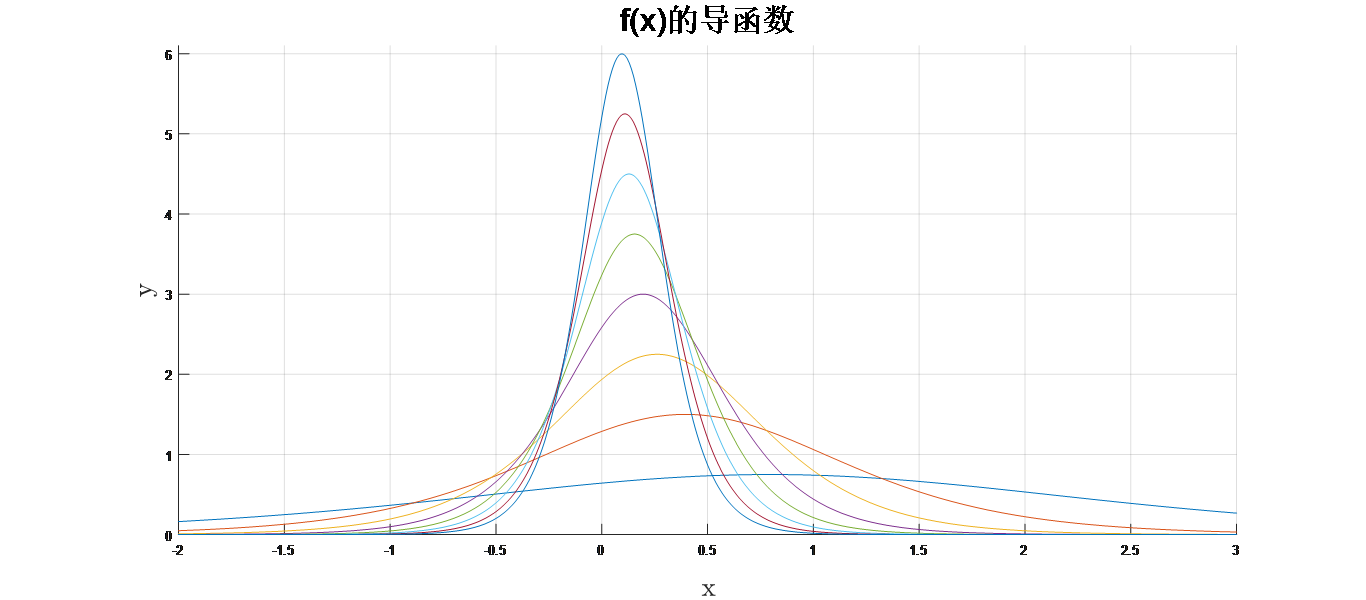展开全文• ## strcpy_s函数

万次阅读 多人点赞 2016-05-06 17:54:13
C++标准中使用了更为安全的strcpy_s函数，让人蛋疼的是为毛不直接更新strcpy函数，非要加_s,可能是为了兼顾旧的工程。不管怎样该用还得用 strcpy_s包含在头文件中，亲测也可，反正就改个名，其定义如下： _...
C++标准中使用了更为安全的strcpy_s函数，让人蛋疼的是为毛不直接更新strcpy函数，非要加_s,可能是为了兼顾旧的工程。不管怎样该用还得用
strcpy_s包含在头文件<string.h>中，亲测<cstring>也可，反正就改个名，其定义如下：
 _ACRTIMP errno_t __cdecl strcpy_s(
_Out_writes_z_(_SizeInBytes) char*       _Destination,
_In_                         rsize_t     _SizeInBytes,
_In_z_                       char const* _Source
);
第一个参数：目标字符串指针
第二个参数：字符串长度，可使用strlen()函数直接求出，切记，在使用strlen()求出字符串长度时，勿忘+1
第三个参数：输入字符串指针
实例如下：
StringBad::StringBad(const char * s)
{
len = strlen(s);	//计算字符串长度
str = new char[len + 1];	//分配存储空间
strcpy_s(str, len+1,s);	//将s中字符串复制到str，最后一个空间为'\0'结束符
num_strings++;
cout << num_strings << ": \"" << str << "\" object created" << endl;
}
展开全文• 在掌握了strcpy函数和strcpy_s函数之后，我们不可避免地会谈到strncpy函数和strncpy_s函数，其实这四个函数的功能几乎一致，就是对两个字符串数组进行复制和赋值，但是具体实现有一点点区别。 首先来说一下strncpy...
在掌握了strcpy函数和strcpy_s函数之后，我们不可避免地会谈到strncpy函数和strncpy_s函数，其实这四个函数的功能几乎一致，就是对两个字符串数组进行复制和赋值，但是具体实现有一点点区别。

首先来说一下strncpy函数。该函数依然还是存在于标准名称空间std内，出现的目的很简单，对于strcpy函数，只能将两个字符串进行完整的复制和赋值，这里就会产生一个实际应用时的问题，如果我们只需要复制某个字符串的前几个字符呢？

其实对于这个问题，我们首先可能会想到使用strcpy_s函数，因为该函数有一个长度，我们在安全函数的基础上将长度表示成我们希望复制的长度，但是实际运行时这样写会出现问题，举例如下：

// strncpy_s.cpp
//

#include "stdafx.h"
#include <iostream>
#include <cstring>

int main()
{
using namespace std;

char str1;
char str2;
cout << "Please enter your favorite lines (Warning: No longer than 100!):\n";
cin.getline(str1, 100);
strcpy_s(str2, 5, str1);
cout << "str1 is " << endl << str1 << endl;
cout << "while str2 is " << endl << str2 << endl;

system("pause");
return 0;
}

对于以上代码，运行结果如下图所示：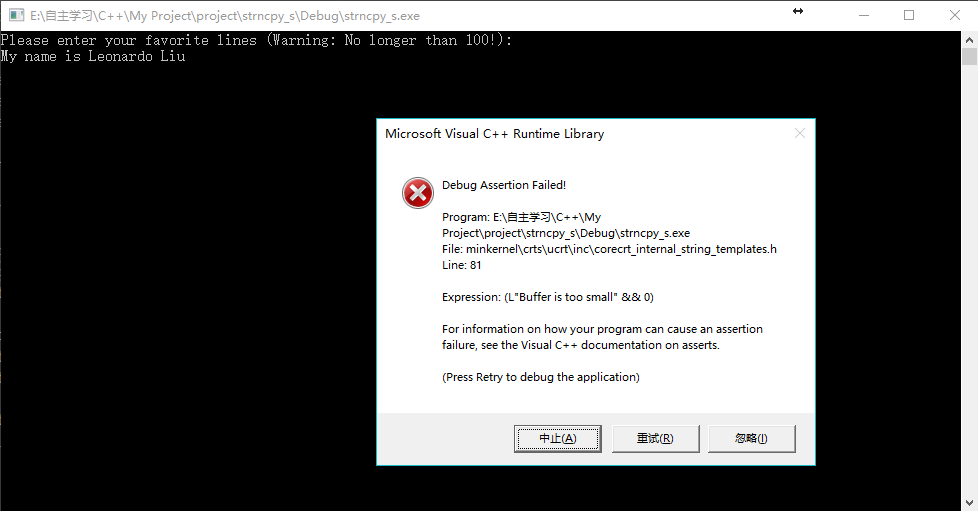由运行结果可知，Buffer is too small，即告诉我们安全函数之所以安全，就是不可以这样操作，不可以把一个长度超过x的字符串数组复制并赋值给长度为x的字符串数组。

于是由此，strncpy函数应运而生。

由于我使用的IDE是Visual Studio 2017，所以只要使用strncpy函数就会报错并提示使用安全版本，所以这里对于基础版本的strncpy函数只做理论介绍。

strncpy函数的原型如下所示：

char * strncpy(char * str2, char * str1, int size);

功能就是复制str1中的内容，赋进str2中，复制的长度由size的数值决定，size的类型不一定是Int，但我们一般来说遇到的长度都是整数，所以这里用int比较简单。

比如说以下语句：

char str1 = "abcd";
char str2 = "leonardo";
strncpy(str2, str1, 3);

因为IDE不支持基础版本函数，所以我没法看运行结果，就文字描述一下。

以上代码运行之后，strncpy函数会将str1的前3个字符的内容复制，赋到str2里，于是str2就变成了‘a’'b'‘c’。

那么对于strncpy_s函数，原型如下所示：

strncpy_s(char * str2, int size2, char * str1, int size1);

这里多了一个长度，就是被复制的str2的长度，我们可以用sizeof(str2)来表示这个长度。

那么改成使用strncpy_s函数之后，上面的代码就可以正确运行了。

// strncpy_s.cpp
//

#include "stdafx.h"
#include <iostream>
#include <cstring>

int main()
{
using namespace std;

char str1 = "abcd";
char str2 = "leonardo";
cout << "str1 is " << endl << str1 << endl;
cout << "str2 is " << endl << str2 << endl;
strncpy_s(str2, sizeof(str2), str1, 3);
cout << "after the copy str2 is " << endl << str2 << endl;

system("pause");
return 0;
}


运行结果如下图所示：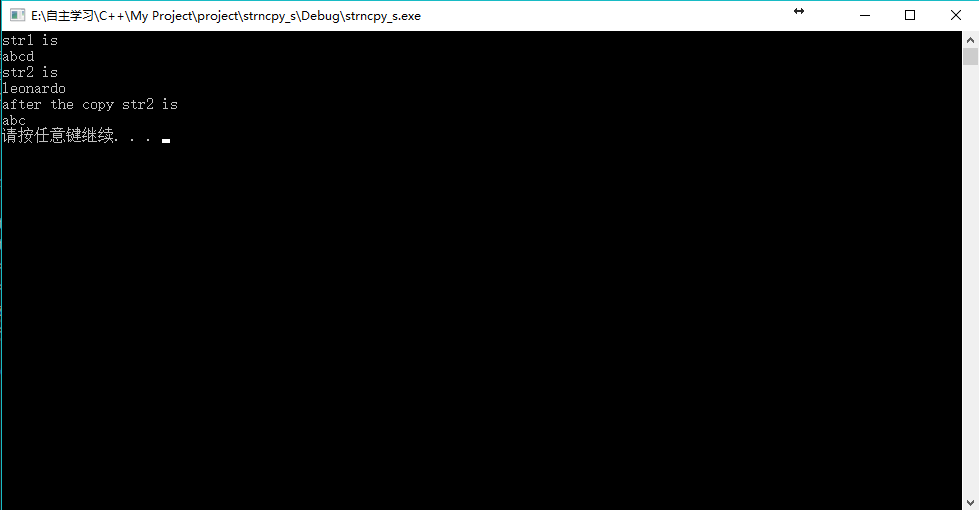于是回到我们最早那个代码，稍作修改使用strncpy_s函数，修改后代码如下：

// strncpy_s.cpp
//

#include "stdafx.h"
#include <iostream>
#include <cstring>

int main()
{
using namespace std;

char str1 = "abcd";
char str2 = "leonardo";
cout << "str1 is " << endl << str1 << endl;
cout << "str2 is " << endl << str2 << endl;
strncpy_s(str2, sizeof(str2), str1, 3);
cout << "after the copy str2 is " << endl << str2 << endl;

char str3;
char str4;
cout << "Please enter your favorite lines (Warning: No longer than 100!):\n";
cin.getline(str3, 100);
strncpy_s(str4, sizeof(str4), str3, 10);
cout << "str3 is " << endl << str3 << endl;
cout << "while str4 is " << endl << str4 << endl;

system("pause");
return 0;
}

对于str3，它本身的长度是100，用户输入可以输入小于100的任意长度的字符，但是str4没有这么长，它的长度只有10，所以只能把str3的前10个字符内容复制给str3。

但是上面这样写还是有点问题，那就是字符串数组的最后一位一定是‘\0'，如果直接复制10位，无法赋进str4，因为这样就把它最后一位的'\0'给覆盖了，就会报错，所以我们修改上面代码的strncpy_s()函数那里，改为

strncpy_s(str4, sizeof(str4), str3, 9);

这样就正确了。

运行结果如下图所示：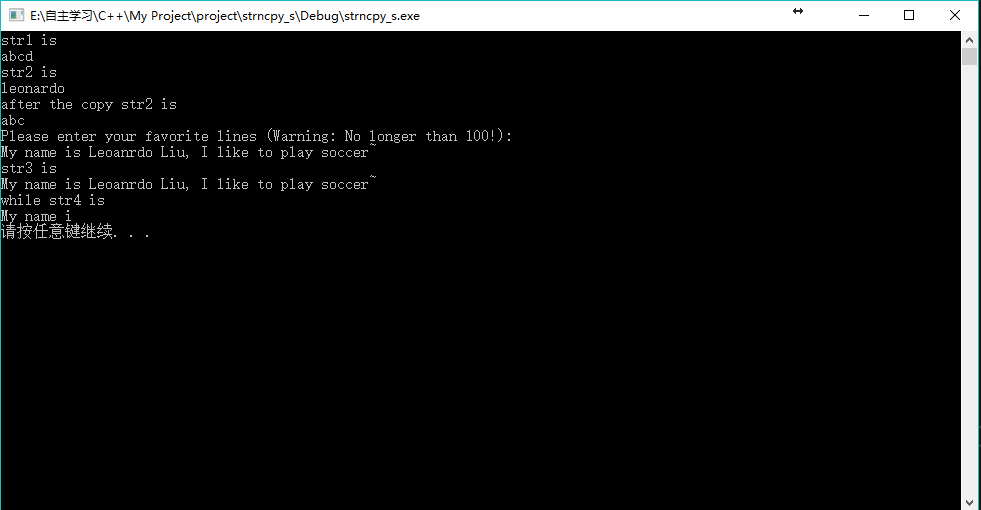于是就完成了我们之前所说的，希望只复制某个字符串数组的一部分给另一个字符串数组的功能了。

其实这个功能还可以延伸，假如说，我们希望复制的字段并不是原字符串最开始的字符怎么办呢，其实也很简单，只需要在strncpy_s函数的第三个参数处进行操作就可以了。

比如说我们希望从第2个字符开始复制，那么第3个参数就由"str3"改写为"str3+1"即可。因为str3是数组，数组名直接使用而不带标号，即是表示地址，所以“str3+1”即是表示str3的地址了，同理"str3+5"即是表示str的地址。

于是我们把上述程序的第23行，即strncpy_s()函数赋值那一句改成如下所示：

strncpy_s(str4, sizeof(str4), str3 + 1, 9);

运行结果如下图：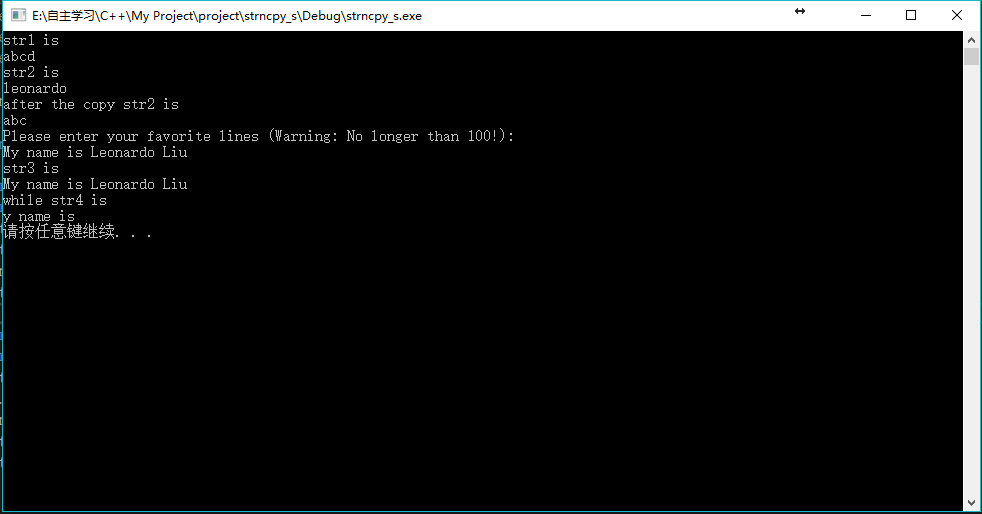从图中可以看出，确实是从第2个字符开始复制的。

那么接下来我们就要考虑，如果希望复制的不是连续的字符，而是分散的，比如说str3,str3,str3和str3这种呢？

这种情况就需要多个strncpy_s语句了，因为每一句只能进行一次复制和赋值，多次才能完成上述任务。

此时我们就需要去操作第1个参数了，即str4，因为str4也是一个数组名表示地址，所以我们这样分散复制时，后面几次复制就需要从str4的str4开始赋值了。

我们来看一下完整代码：

// strncpy_s.cpp
//

#include "stdafx.h"
#include <iostream>
#include <cstring>

int main()
{
using namespace std;

char str1 = "abcd";
char str2 = "leonardo";
cout << "str1 is " << endl << str1 << endl;
cout << "str2 is " << endl << str2 << endl;
strncpy_s(str2, sizeof(str2), str1, 3);
cout << "after the copy str2 is " << endl << str2 << endl;
cout << endl;

char str3;
char str4;
cout << "Please enter your favorite lines (Warning: No longer than 100!):\n";
cin.getline(str3, 100);
cout << endl;
strncpy_s(str4, sizeof(str4), str3 + 2, 1);
strncpy_s(str4 + 1, sizeof(str4) - 1, str3 + 5, 1);
strncpy_s(str4 + 2, sizeof(str4) - 2, str3 + 8, 2);
cout << "str3 is " << endl << str3 << endl;
cout << "while str4 is " << endl << str4 << endl;

system("pause");
return 0;
}

上述代码的第25,26,27三行都是在进行strncpy_s函数的复制，可以看到我们分了3次复制，因为str3，str3都只能单独复制，因为不连续，而str3和str3可以联合复制，因为连续。

上述代码运行结果如下图所示：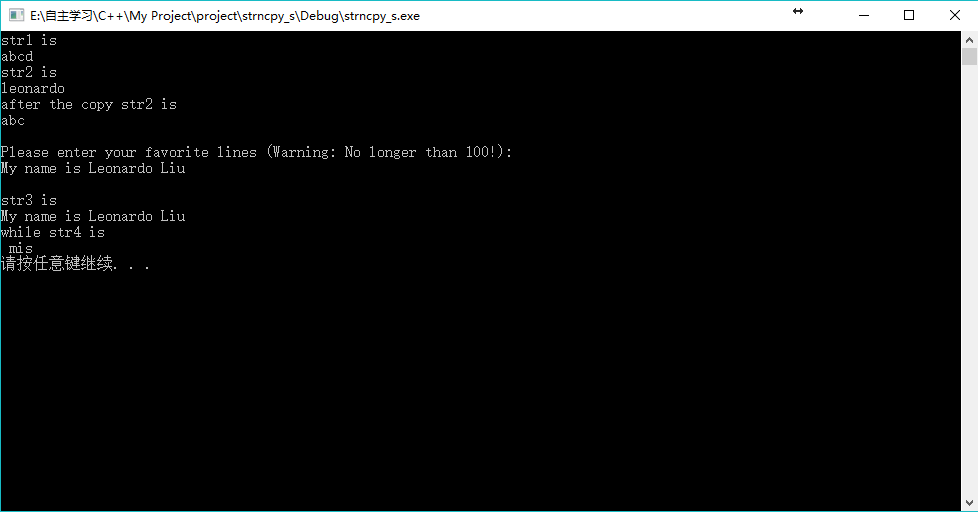从图中可以看到，str3是‘ ’，str3是'm'，str3是'i',str3是's'。

所以这样我们就完成了分散复制的功能了。

目前我掌握的有关strncpy函数和strncpy_s函数的使用及注意事项就是这样，以后继续学习遇到新的知识了会持续补充。

展开全文strncpy
• 文章目录什么叫S函数用MATLAB语言编写S函数(1)主函数(2)子函数S函数的应用(1)定义s函数1.主函数2.初始化子函数3.输出子函数完整代码（2）在Simulink模型中使用S函数 B站av52613760/p52 什么叫S函数 s函数是系统函数...MATLAB
• ## S函数模板

千次阅读 2017-03-27 16:52:54
本文为simulink中s函数模板的翻译版。可直接拷入m文件编辑。matlab
• ## simulink之S函数

千次阅读 2018-08-11 08:40:47
s函数是system Function的简称，用它来写自己的simulink模块。（够简单吧，^_^，详细的概念介绍大伙看帮助吧）可以用matlab、C、C++、Fortran、Ada等语言来写，这儿我只介绍怎样用matlab语言来写吧（主要是它比较...
• ## simulink中S函数格式

千次阅读 2019-04-26 20:39:24
s函数可以利用matlab的丰富资源，而不仅仅局限于simulink提供的模块，而用c或c++等语言写的s函数还可以实现对硬件端口的操作。 S函数格式 在matlab的workspace里打edit sfuntmpl，就可以看到matlab自己提供的s函数...
• ## matlab中S函数的概念及使用

万次阅读 多人点赞 2018-04-08 21:03:31
S函数即系统函数System Function的意思，为什么要使用S函数呢？是因为在研究中，有时需要用到复杂的算法设计等，而这些算法因为其复杂性不适合用普通的Simulink模块来搭建，即matlab所提供的Simulink模块不能满足...matlab simulink
• S函数是什么？有什么用？ S函数(Sigmoid function)是BP神经网络中常用的非线性作用函数，即sigmoid函数，公式是f(x)=1/(1+e-x)(-x是幂数) Sigmoid函数又分为Log-Sigmoid函数和Tan-Sigmoid函数。 ...
• 文章目录文章来源和摘要S函数的编写格式和运行步骤simulink模型结构S函数模型初始化部分代码理解S函数模型更新部分S函数模型输出部分S函数完整代码附录 文章来源和摘要 S函数的编写格式和运行步骤 S函数相当于...
• ## s函数小实例

千次阅读 2018-07-14 16:16:53
采用S函数实现y=kx+b。①主函数function [sys,x0,str,ts]=timekb(t,x,u,flag,k,b)switch flag case 0 [sys,x0,str,ts]=mdlInitializeSizes; %初始化 case 3 sys=mdlOutputs(t,x,u,k,b); %计算输出量 ca...
• 带双参数的双极S函数sigmod(x,α\alpha,β\beta)神经网络
• Sigmoid函数，即f(x)=1/(1+e-x)。神经元的非线性作用函数。（-x是幂数） 人工神经网络的学习算法-BP算法  神经网络的学习是基于一组样本进行的，它包括输入和输出（这里用期望输出表示），输入和输出有多少...
• 文章目录S函数S函数类型S函数的组成及执行顺序编写S函数Level1 M S函数Level2 M S函数C Mex S函数mdlInitializeSizesC Mex S函数的实例 S函数 当Simulink默认提供的模块不能够满足用户的需求时，用户可以通过S函数...Simulink TLC
• S函数的相关概念与写法，直接在帮助文件中搜：【MATLAB S-Functions Create custom blocks defined】、【S-Function Concepts】等 S函数模块可以从下图中拖出来： 图 1 其中S-Function是正宗的S函数模块，...
• 论VS2017中的scanf函数和scanf_s函数的区别 ** 在visual studio 2017中格式化输入函数不同于其他c/c++编译器使用scanf,而是使用scanf_s。 scanf_s相比较于scanf来说更安全，因为使用scanf_s函数需要设定有一个缓冲...
• S函数即系统函数System Function的意思，在研究中，有时需要用到复杂的算法设计等，而这些算法因为其复杂性不适合用普通的Simulink模块来搭建，即matlab所提供的Simulink模块不能满足用户的需求，需要用编程的形式...
• ## scala中的s函数

千次阅读 2016-11-07 11:14:10
//s函数的应用 val name="Tom" s"Hello,$name"//Hello,Tom s"1+1=${1+1}"//1+1=2
• ## matlab中s函数

千次阅读 2011-12-04 19:55:31
matlab中s函数编写s函数是system Function的简称，用它来写自己的simulink模块。（够简单吧，^_^，详细的概念介绍大伙看帮助吧）可以用matlab、C、C++、Fortran、Ada等语言来写，这儿我只介绍怎样用matlab语言来写吧...matlab function fortran 语言 任务
• ## VS中的scanf_s函数和scanf

万次阅读 多人点赞 2018-06-15 18:03:22
ANSI C中没有scanf_s(),只有scanf()，scanf()在读取时...目前最新的c11标准中已经将scanf_s函数“转正”了。 http://msdn.microsoft.com/zh-cn/library/w40768et%28VS.80%29.aspx 如果想继续使用scanf这个不安全的VS
• TP框架中S函数使用步骤： //初始化 type为缓存方式;prefix为缓存前缀标示,也就是名字;expire为生存周期  //我这里设置缓存方式为xcache,名字ticket,周期7100秒 cache=S(array(′type′=&gt;′xcache′,′prefix...
• 文章目录(〇) 前言(一) 编写S函数(二)S函数的应用：  （1）定义S函数        ①初始化主函数        ②初始化子函数&...matlab simulink
• Matlab允许你使用以下五种方式之一来实现S函数： 　 A Level-1 M-file S-function provides a simple M interface to interact with a small portion of the S-function API. Level-2 M-file S-functions supersede...matlab
• ## s函数诠释

千次阅读 2005-04-26 00:59:00
这是我摸索s函数的一些心得，与大伙分享。 s函数是system Function的简称，用它来写自己的simulink模块。（够简单吧，^_^，详细的概念介绍大伙看帮助吧）可以用matlab、C、C++、Fortran、Ada等语言来写，这儿我只...matlab function fortran 语言 c++
• ## Matlab S函数编写

千次阅读 2009-04-10 19:20:00
s函数是system Function的简称，用它来写自己的simulink模块。（够简单吧，^_^，详细的概念介绍大伙看帮助吧）可以用matlab、C、C++、Fortran、Ada等语言来写，这儿我只介绍怎样用matlab语言来写吧（主要是它比较...matlab function fortran 语言 任务
• ## sprintf_s函数用法

万次阅读 2017-10-26 16:08:57
sprintf_s是一个函数，其函数功能是将数据格式化输出到字符串。sprintf_s对于格式化string中的格式化的字符的有效性进行了检查，sprintf_s也携带着接收格式化字符串的缓冲区的大小。 sprintf_s将格式化字符串存到...
• 1.thinkphp中的s函数是文件缓存吗？这个s函数文件缓存是静态缓存吗？ 2.memcache是动态缓存？php 缓存...